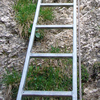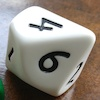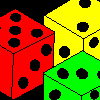# Resources tagged with: Mathematical reasoning & proof

Filter by: Content type:
Age range:
Challenge level:

### There are 96 results

Broad Topics > Thinking Mathematically > Mathematical reasoning & proof### The Genie in the Jar

##### Age 11 to 14Challenge Level

This jar used to hold perfumed oil. It contained enough oil to fill granid silver bottles. Each bottle held enough to fill ozvik golden goblets and each goblet held enough to fill vaswik crystal. . . .### Largest Product

##### Age 11 to 14Challenge Level

Which set of numbers that add to 10 have the largest product?### Always, Sometimes or Never? Number

##### Age 7 to 11Challenge Level

Are these statements always true, sometimes true or never true?### Square Subtraction

##### Age 7 to 11Challenge Level

Look at what happens when you take a number, square it and subtract your answer. What kind of number do you get? Can you prove it?### Gabriel's Problem

##### Age 11 to 14Challenge Level

Gabriel multiplied together some numbers and then erased them. Can you figure out where each number was?### Three Neighbours

##### Age 7 to 11Challenge Level

Look at three 'next door neighbours' amongst the counting numbers. Add them together. What do you notice?### One O Five

##### Age 11 to 14Challenge Level

You can work out the number someone else is thinking of as follows. Ask a friend to think of any natural number less than 100. Then ask them to tell you the remainders when this number is divided by. . . .### Aba

##### Age 11 to 14Challenge Level

In the following sum the letters A, B, C, D, E and F stand for six distinct digits. Find all the ways of replacing the letters with digits so that the arithmetic is correct.### What Numbers Can We Make?

##### Age 11 to 14Challenge Level

Imagine we have four bags containing a large number of 1s, 4s, 7s and 10s. What numbers can we make?### Take Three Numbers

##### Age 7 to 11Challenge Level

What happens when you add three numbers together? Will your answer be odd or even? How do you know?### Eleven

##### Age 11 to 14Challenge Level

Replace each letter with a digit to make this addition correct.### Take One Example

##### Age 5 to 11

This article introduces the idea of generic proof for younger children and illustrates how one example can offer a proof of a general result through unpacking its underlying structure.### Even So

##### Age 11 to 14Challenge Level

Find some triples of whole numbers a, b and c such that a^2 + b^2 + c^2 is a multiple of 4. Is it necessarily the case that a, b and c must all be even? If so, can you explain why?##### Age 11 to 14Challenge Level

Make a set of numbers that use all the digits from 1 to 9, once and once only. Add them up. The result is divisible by 9. Add each of the digits in the new number. What is their sum? Now try some. . . .##### Age 7 to 14Challenge Level

I added together some of my neighbours' house numbers. Can you explain the patterns I noticed?### What Numbers Can We Make Now?

##### Age 11 to 14Challenge Level

Imagine we have four bags containing numbers from a sequence. What numbers can we make now?##### Age 5 to 11Challenge Level

Who said that adding couldn't be fun?### Reasoning: the Journey from Novice to Expert (article)

##### Age 5 to 11

This article for primary teachers suggests ways in which we can help learners move from being novice reasoners to expert reasoners.### Always, Sometimes or Never?

##### Age 5 to 11Challenge Level

Are these statements relating to odd and even numbers always true, sometimes true or never true?### What Do You Need?

##### Age 7 to 11Challenge Level

Four of these clues are needed to find the chosen number on this grid and four are true but do nothing to help in finding the number. Can you sort out the clues and find the number?### Reasoning: Identifying Opportunities (article)

##### Age 5 to 11

In this article for primary teachers we consider in depth when we might reason which helps us understand what reasoning 'looks like'.### Less Is More

##### Age 5 to 11Challenge Level

Use your knowledge of place value to try to win this game. How will you maximise your score?### Chocolate Maths

##### Age 11 to 14Challenge Level

Pick the number of times a week that you eat chocolate. This number must be more than one but less than ten. Multiply this number by 2. Add 5 (for Sunday). Multiply by 50... Can you explain why it. . . .##### Age 11 to 14Challenge Level

Powers of numbers behave in surprising ways. Take a look at some of these and try to explain why they are true.### Making Pathways

##### Age 7 to 11Challenge Level

Can you find different ways of creating paths using these paving slabs?### Take Three from Five

##### Age 11 to 16Challenge Level

Caroline and James pick sets of five numbers. Charlie chooses three of them that add together to make a multiple of three. Can they stop him?### Cows and Sheep

##### Age 7 to 11Challenge Level

Use your logical reasoning to work out how many cows and how many sheep there are in each field.### Go Forth and Generalise

##### Age 11 to 14

Spotting patterns can be an important first step - explaining why it is appropriate to generalise is the next step, and often the most interesting and important.### Elevenses

##### Age 11 to 14Challenge Level

How many pairs of numbers can you find that add up to a multiple of 11? Do you notice anything interesting about your results?### Online

##### Age 7 to 11Challenge Level

A game for 2 players that can be played online. Players take it in turns to select a word from the 9 words given. The aim is to select all the occurrences of the same letter.##### Age 7 to 11Challenge Level

Three dice are placed in a row. Find a way to turn each one so that the three numbers on top of the dice total the same as the three numbers on the front of the dice. Can you find all the ways to do. . . .### Sticky Numbers

##### Age 11 to 14Challenge Level

Can you arrange the numbers 1 to 17 in a row so that each adjacent pair adds up to a square number?### True or False?

##### Age 7 to 11Challenge Level

Without doing lots of calculations, can you decide which of these number sentences are true? How do you know?### Dicing with Numbers

##### Age 11 to 14Challenge Level

In how many ways can you arrange three dice side by side on a surface so that the sum of the numbers on each of the four faces (top, bottom, front and back) is equal?### Tis Unique

##### Age 11 to 14Challenge Level

This addition sum uses all ten digits 0, 1, 2...9 exactly once. Find the sum and show that the one you give is the only possibility.### 9 Weights

##### Age 11 to 14Challenge Level

You have been given nine weights, one of which is slightly heavier than the rest. Can you work out which weight is heavier in just two weighings of the balance?### Interactive Balance

##### Age 5 to 11Challenge Level

In this simulation of a balance, you can drag numbers and parts of number sentences on to the trays. Have a play!### More Mathematical Mysteries

##### Age 11 to 14Challenge Level

Write down a three-digit number Change the order of the digits to get a different number Find the difference between the two three digit numbers Follow the rest of the instructions then try. . . .### Unit Fractions

##### Age 11 to 14Challenge Level

Consider the equation 1/a + 1/b + 1/c = 1 where a, b and c are natural numbers and 0 < a < b < c. Prove that there is only one set of values which satisfy this equation.### More Number Pyramids

##### Age 11 to 14Challenge Level

When number pyramids have a sequence on the bottom layer, some interesting patterns emerge...### Logic

##### Age 7 to 14

What does logic mean to us and is that different to mathematical logic? We will explore these questions in this article.### More Number Sandwiches

##### Age 11 to 16Challenge Level

When is it impossible to make number sandwiches?### Seven Squares - Group-worthy Task

##### Age 11 to 14Challenge Level

Choose a couple of the sequences. Try to picture how to make the next, and the next, and the next... Can you describe your reasoning?### Always, Sometimes or Never? Shape

##### Age 7 to 11Challenge Level

Are these statements always true, sometimes true or never true?### Tourism

##### Age 11 to 14Challenge Level

If you can copy a network without lifting your pen off the paper and without drawing any line twice, then it is traversable. Decide which of these diagrams are traversable.### Guess What?

##### Age 7 to 11Challenge Level

Can you find out which 3D shape your partner has chosen before they work out your shape?### Sweetie Box

##### Age 5 to 11Challenge Level

Max and Bryony both have a box of sweets. What do you know about the number of sweets they each have?### Advent Calendar 2011 - Secondary

##### Age 11 to 18Challenge Level

Advent Calendar 2011 - a mathematical activity for each day during the run-up to Christmas.### Children at Large

##### Age 11 to 14Challenge Level

There are four children in a family, two girls, Kate and Sally, and two boys, Tom and Ben. How old are the children?### Top-heavy Pyramids

##### Age 11 to 14Challenge Level

Use the numbers in the box below to make the base of a top-heavy pyramid whose top number is 200.